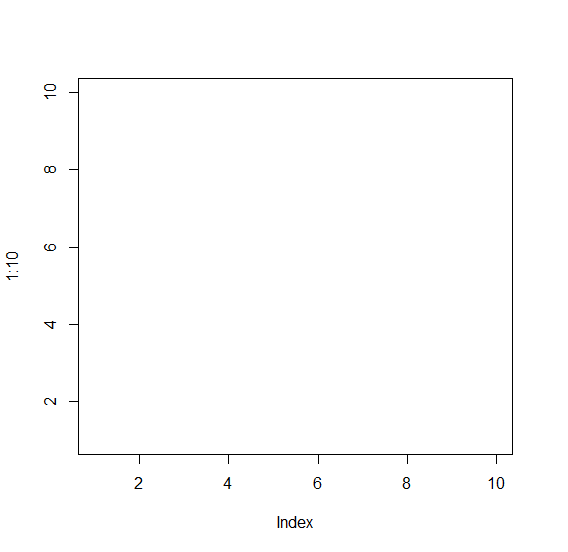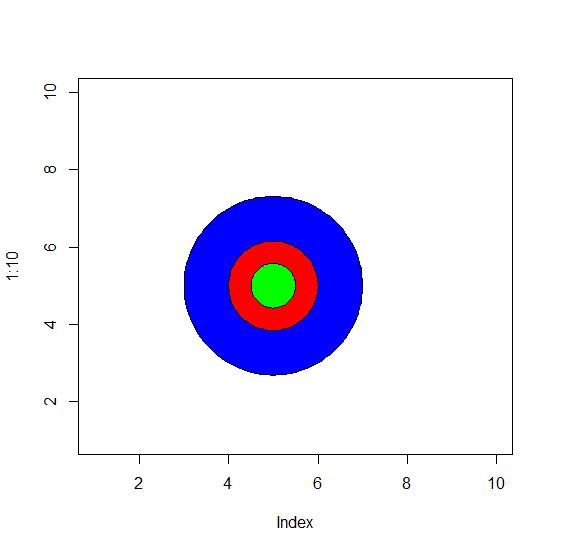# How to draw concentric circles in R?

R ProgrammingServer Side ProgrammingProgramming

#### Artificial Intelligence : The Future Of Programming

15 Lectures 54 mins

#### Beyond Basic Programming - Intermediate Python

Most Popular

36 Lectures 3 hours

#### C Programming from scratch- Master C Programming

Best Seller

60 Lectures 8 hours

To draw concentric circles, we can use draw.circle function of plotrix package but firstly, we would need to create a blank graph with plot function as shown below.

For Example, we can create three concentric circles at position X=5 and Y=5 having radius of 1, 2, and 3 by using the below command −

draw.circle(5,5,c(3,2,1),col=c("blue","red","green"))

## Example

To create three concentric circles at position X=5 and Y=5 having radius of 1, 2, and 3 add the following code to the above snippet −

plot(1:10,type="n")

## Output

If you execute all the above given snippets as a single program, it generates the following Output −To create three concentric circles at position X=5 and Y=5 having radius of 1, 2, and 3 on the above created data frame, add the following code to the above snippet −

plot(1:10,type="n")
library(plotrix)
draw.circle(5,5,c(2,1,0.5),col=c("blue","red","green"))

## Output

If you execute all the above given snippets as a single program, it generates the following Output −